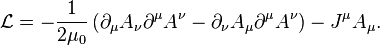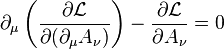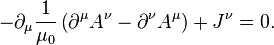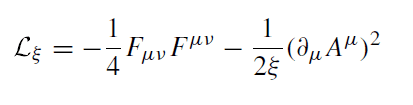# QFT - Derivative in Equation of Motion

## Homework Statement

As part of a problem, I need to derive the EOM for a generalized Lagrangian. Before I get there, I'm trying to refresh myself on exactly how these derivatives work because the notation is so bizarre. I am trying to follow a simple example I found online:## Homework Equations

The E-L eq:## The Attempt at a Solution

The actual solution is:I totally understand the second term, but the first term is bothering me (namely the part INSIDE the parentheses where there are all upper indices). How, pray tell, do we end up with all upper indices? Is it because the mu and nu are just dummy indices, and all that matters is that they are both lower and different? If so, then I can see how you end up with all upper indices. But, why are the upper indices on the right changed (mu <---> nu) if that's the case? Just to distinguish is from the left term so that they don't cancel? QFT is not my favorite subject...

Once I get this, I think I can tackle the actual problem I am solving, which is to find the EOM of the following Lagrangian:Thank you!